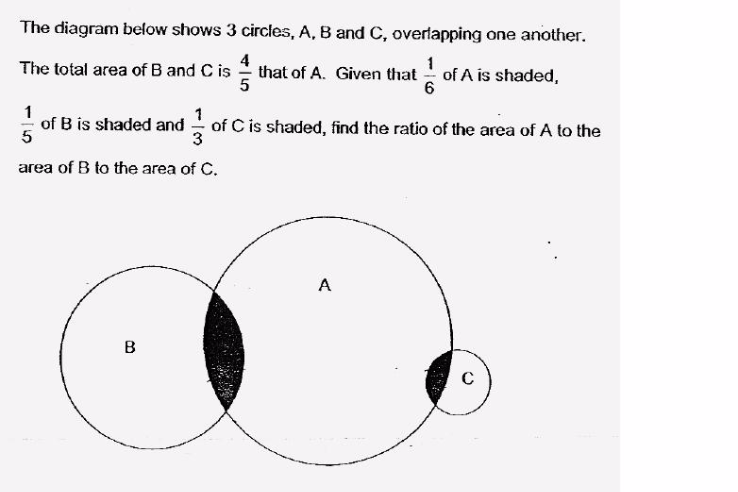# QuestionThank You for your time in helping to solve this problem.Trying to figure out without using Algebra.

Ref

hi tianzhu.

the area refers to the white space labeled A,B and C, or the area of the circle A, B, C ?

lizawa

Hi lizawa
I believe it refers to the areas of circle A,B and C.

Total area of cirlces
(B+C) : A
= 4:5
= 24:30

1/6 of A shaded -> 1/6 * 30 = 5 units shaded
unshaded A -> 30 units – 5 units = 25 units
unshaded (B+C) -> 24 – 5 = 19 units

Can draw model here, to see clearer.
B has 5 units, C has 3 units. Size of units in B and C are different.
5 units of B + 3 units of C -> 24

1 unit of B + 1unit of C -> 5
3 units of B + 3 units of C ->15

2 units of B -> 24 – 15 = 9
unshaded B = 4 units of B -> 9 * 2 = 18

2 units of C -> 24 – 18 – 5 = 1
unshaded C = 2 units = 1

A:B:C
= 25:18:1

Hi lizawa

Thanks
The answer listed in the worksheet is 20:15:1

then, in that case, it’s asking for the total area of A : B:C

Extension to the above solution,